Successfully reported this slideshow.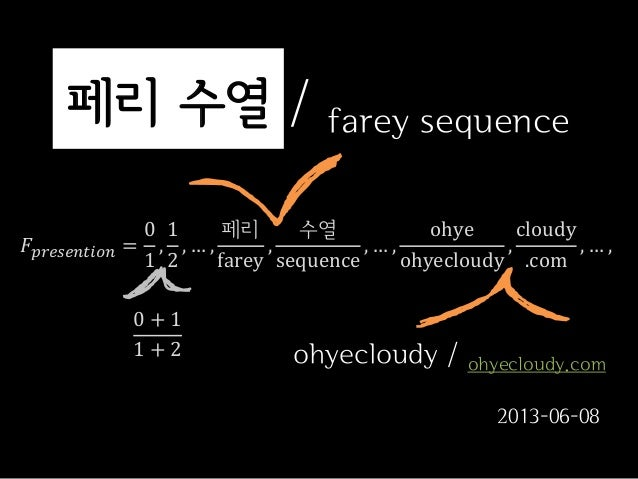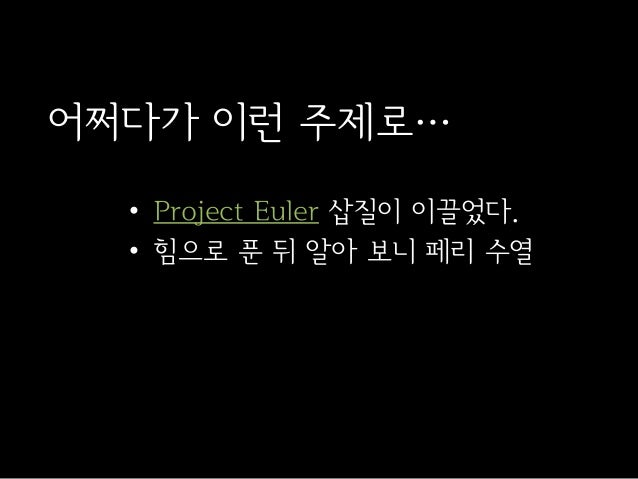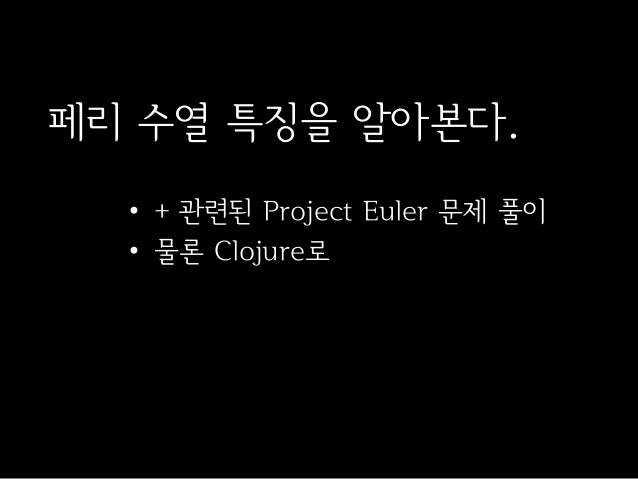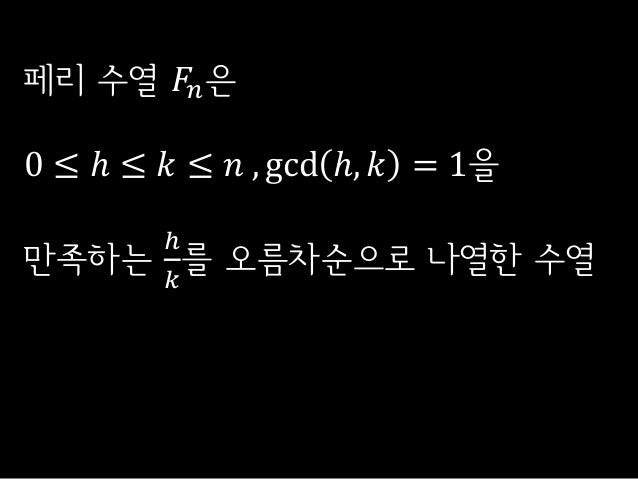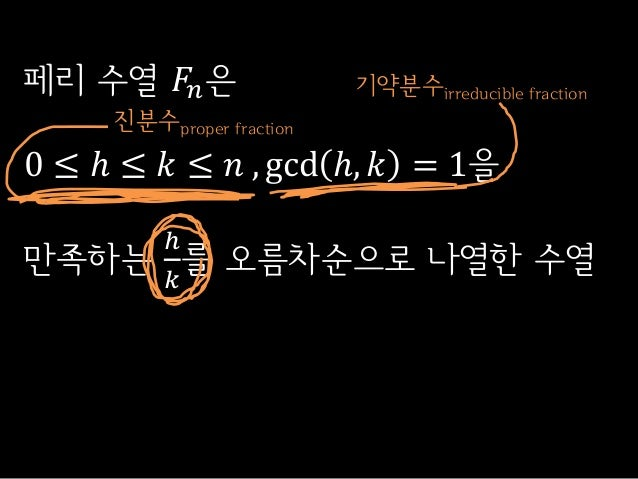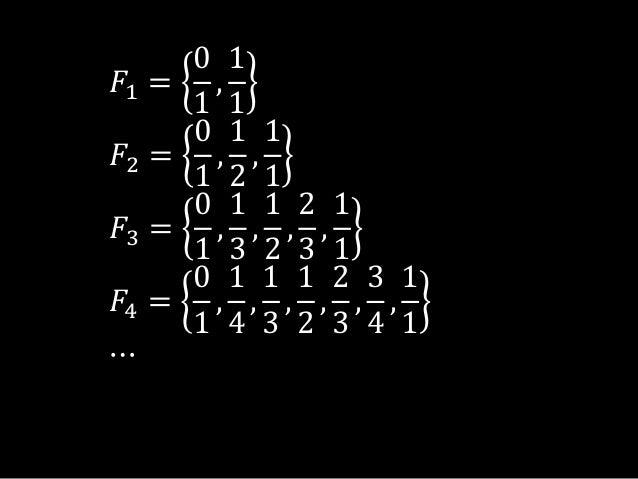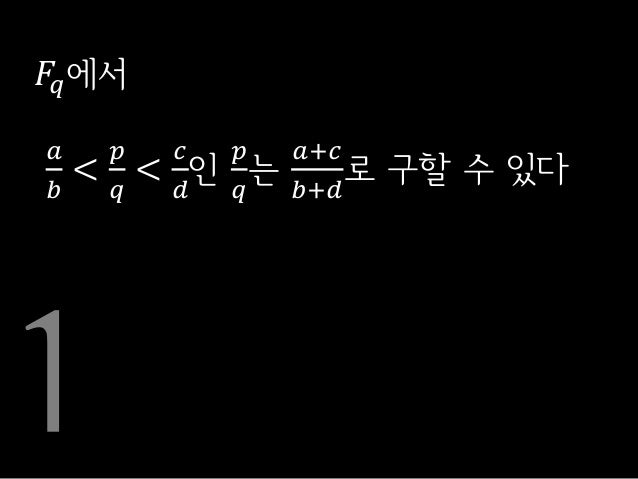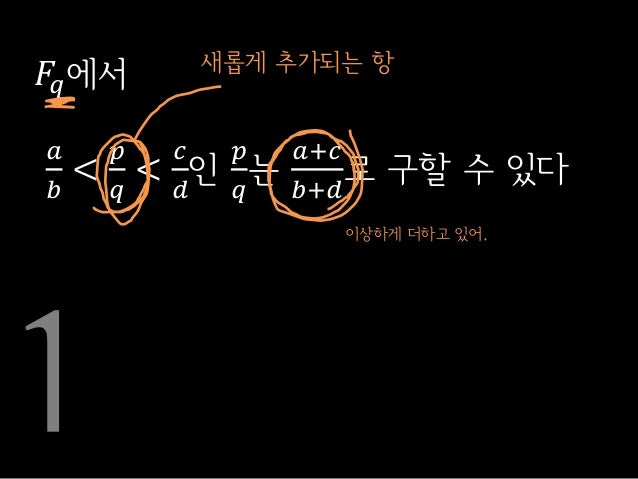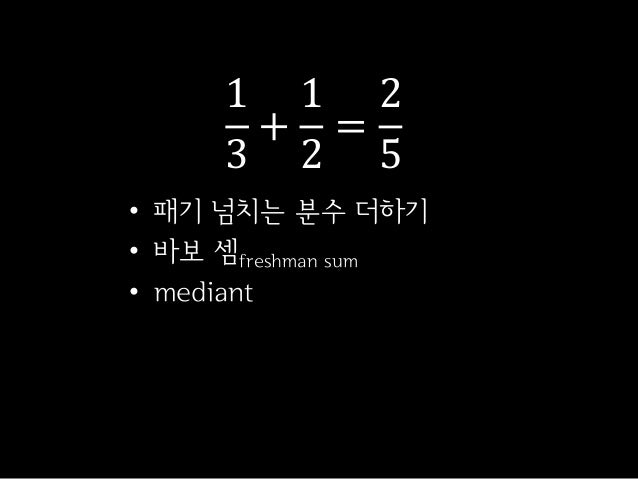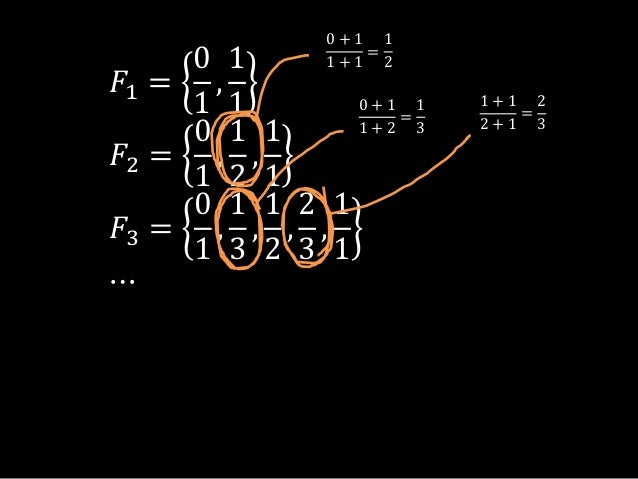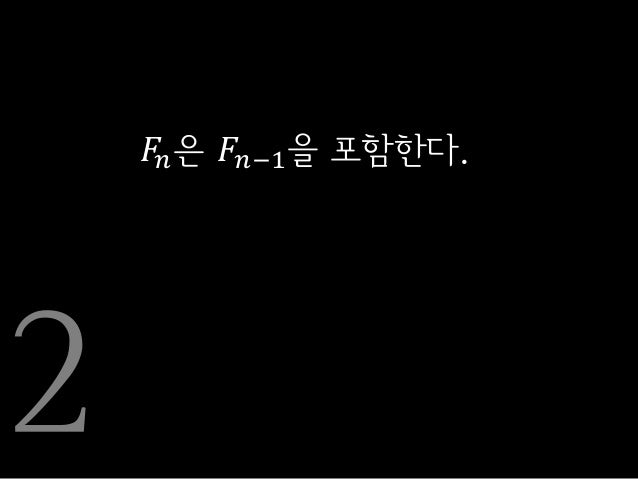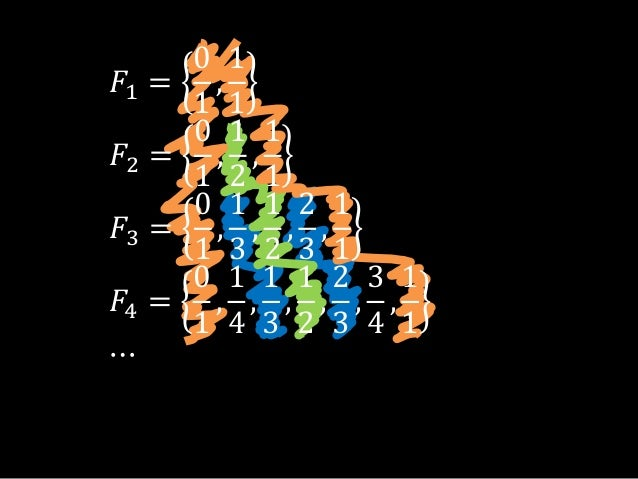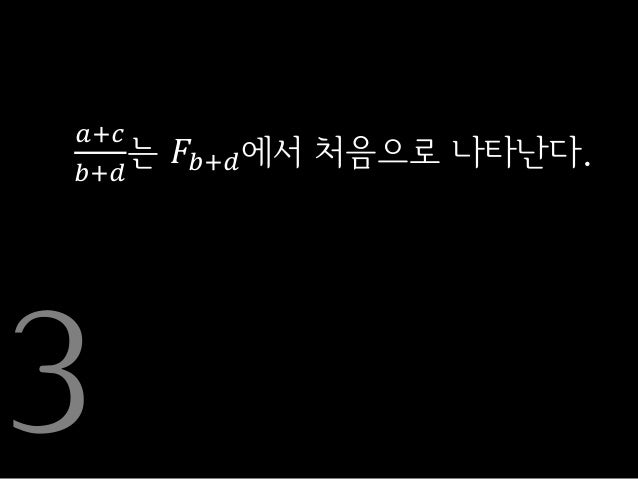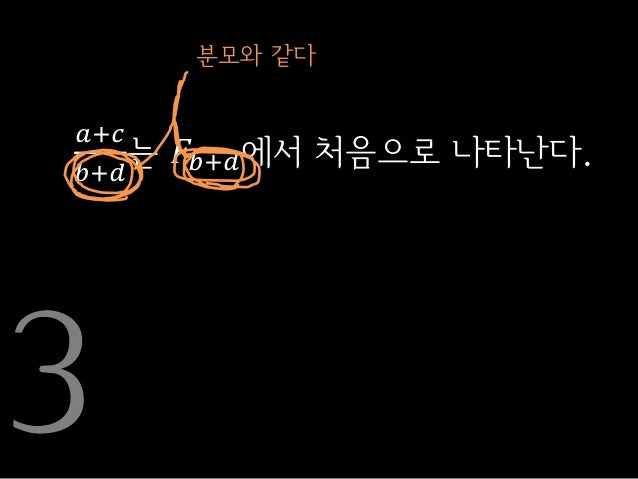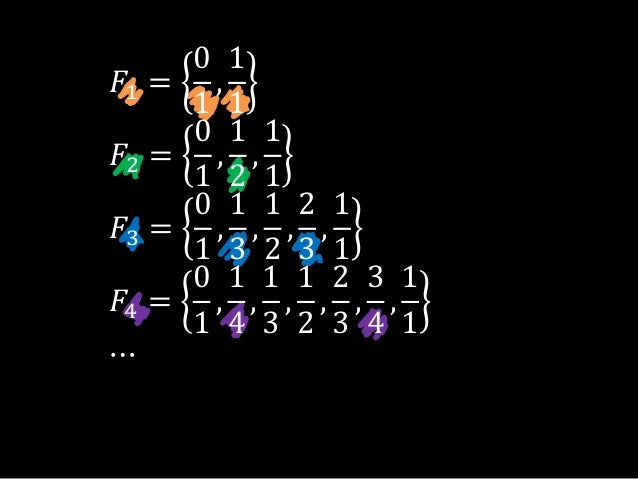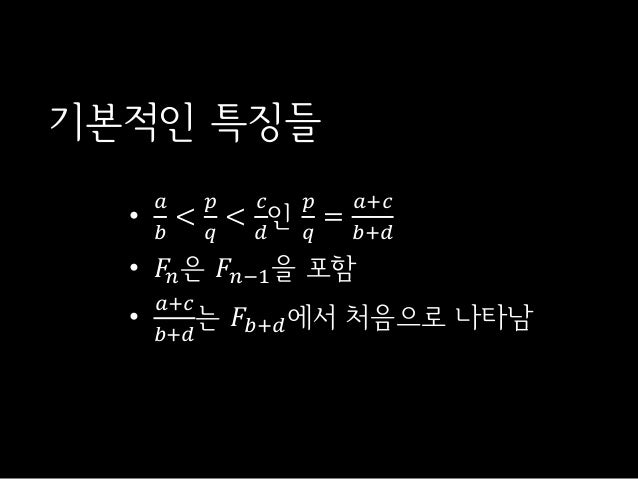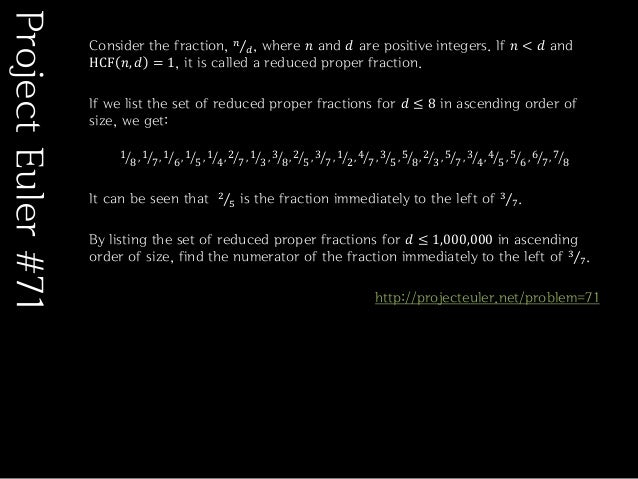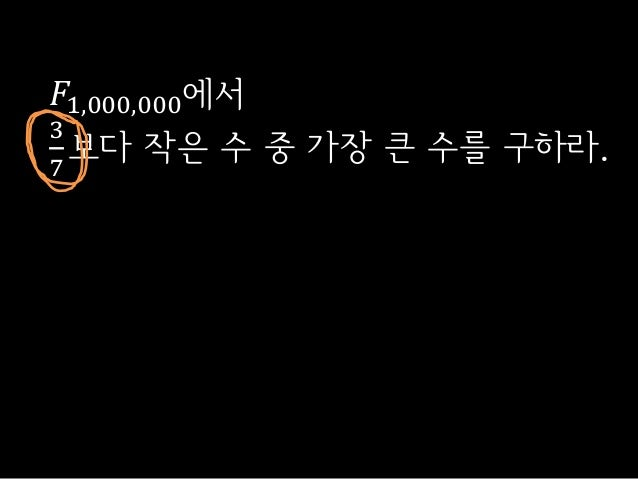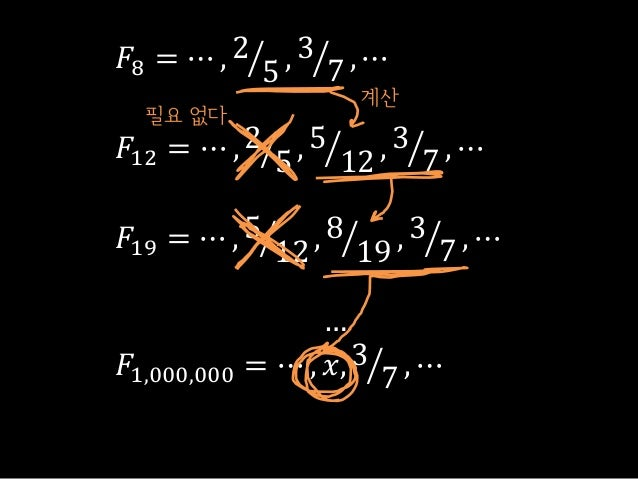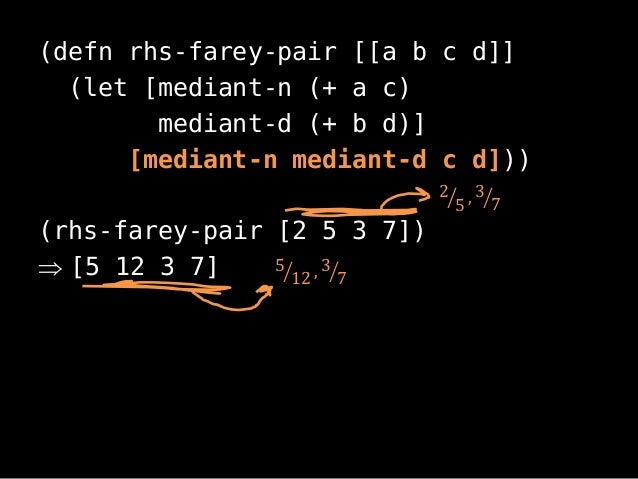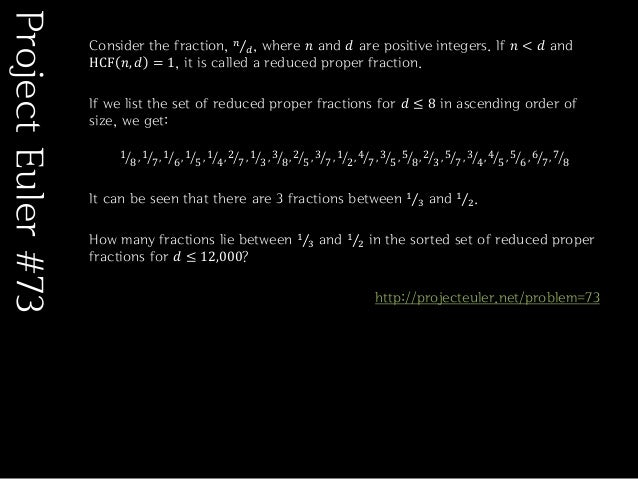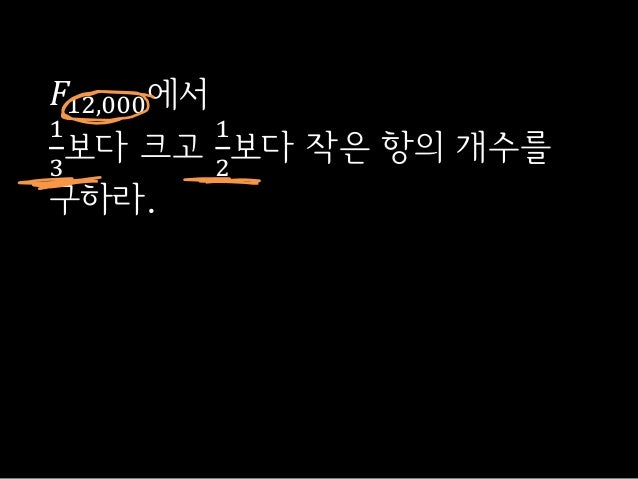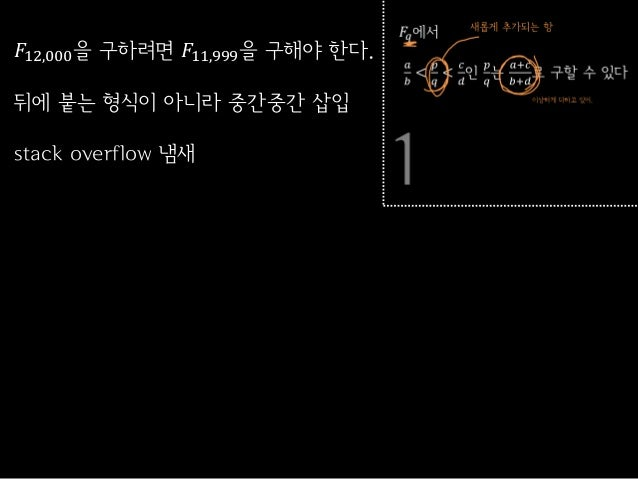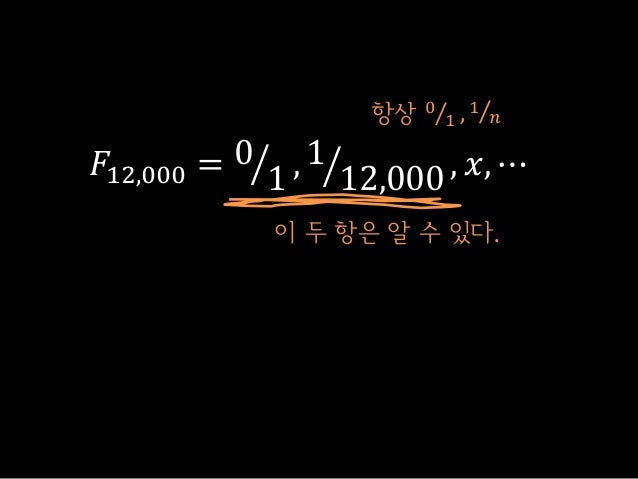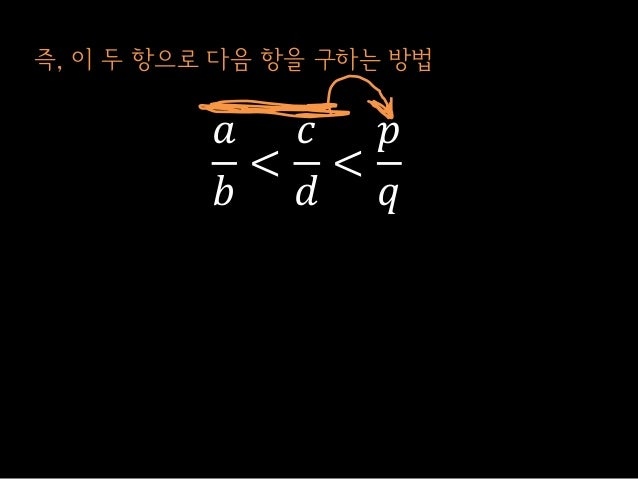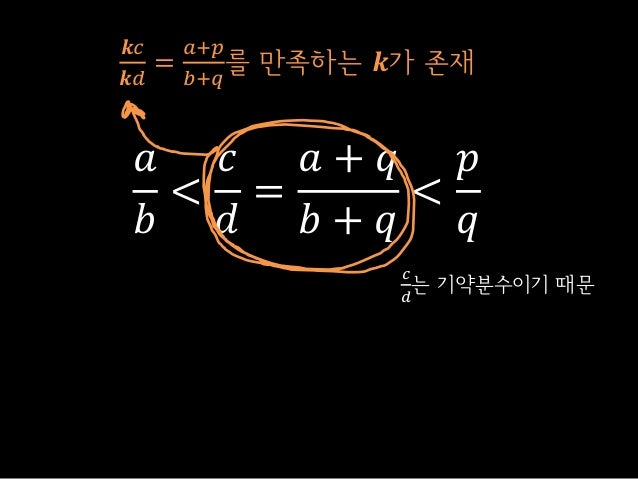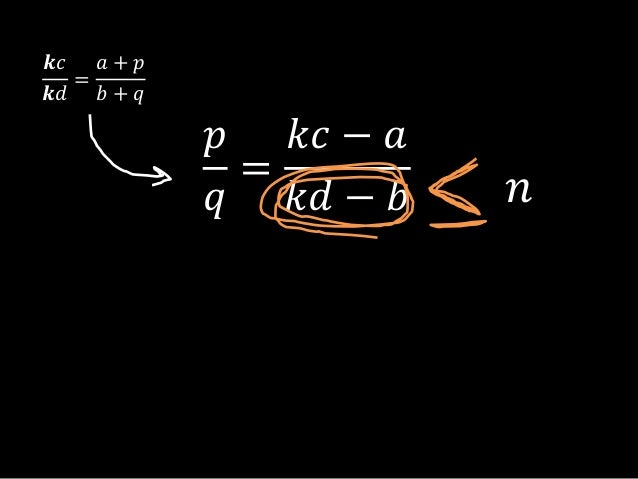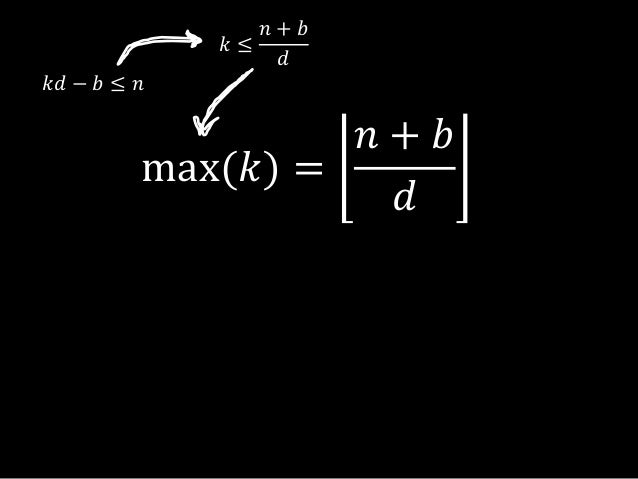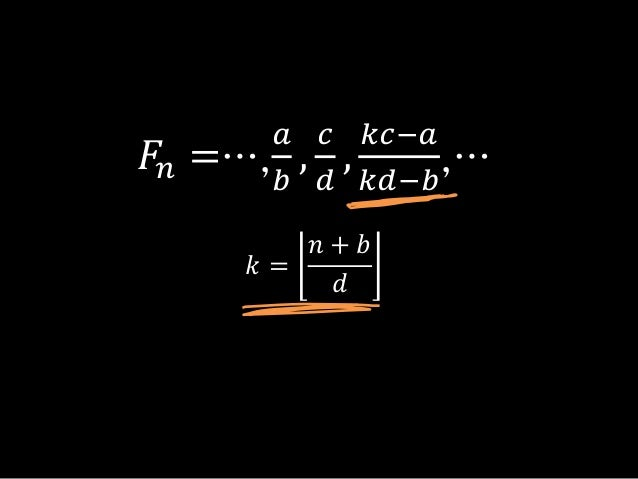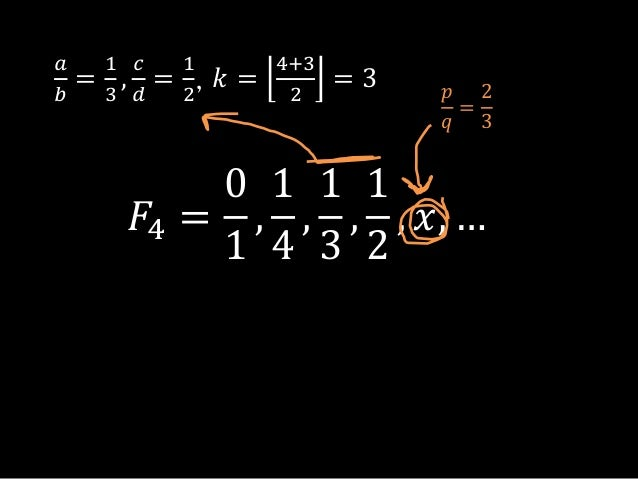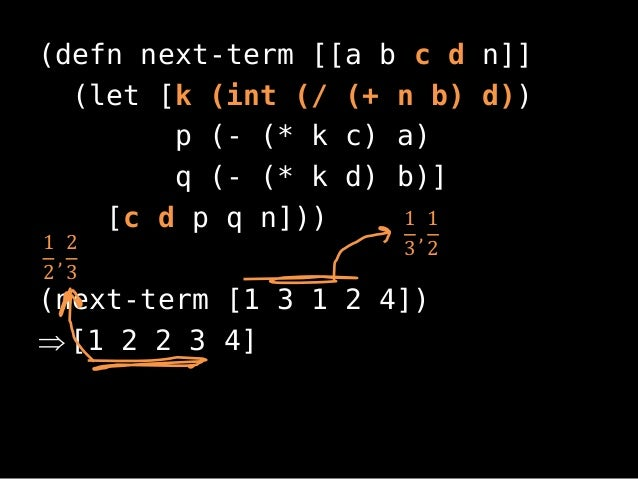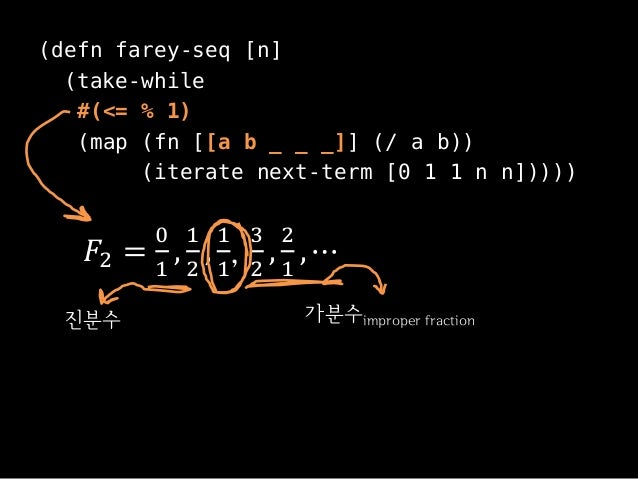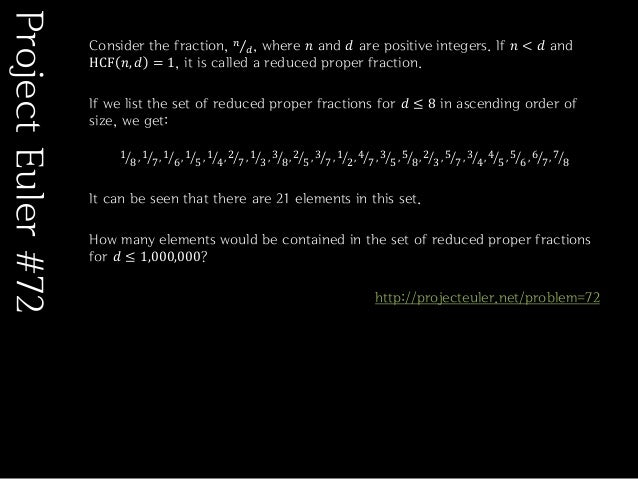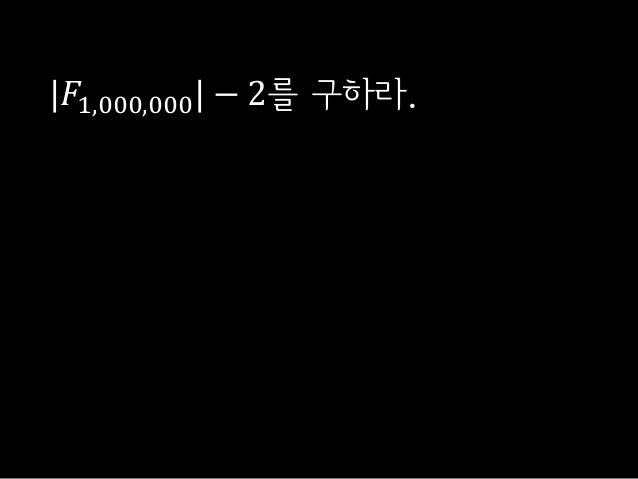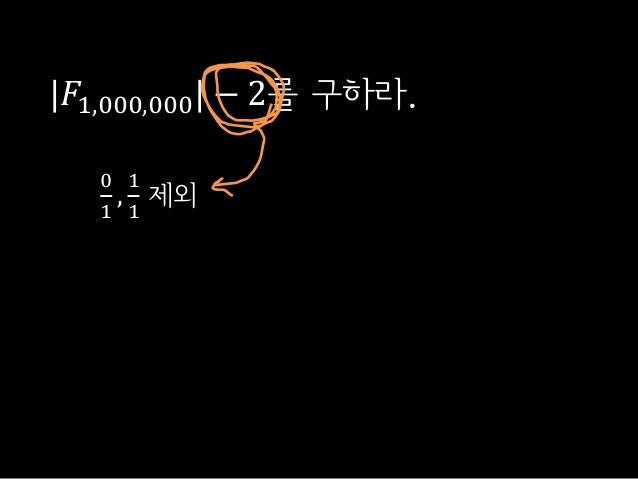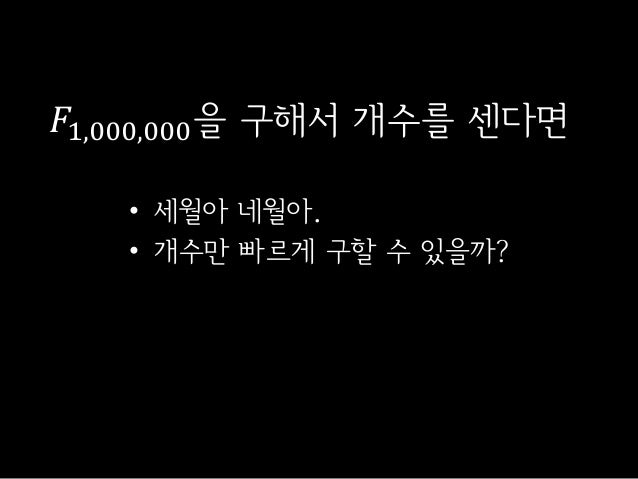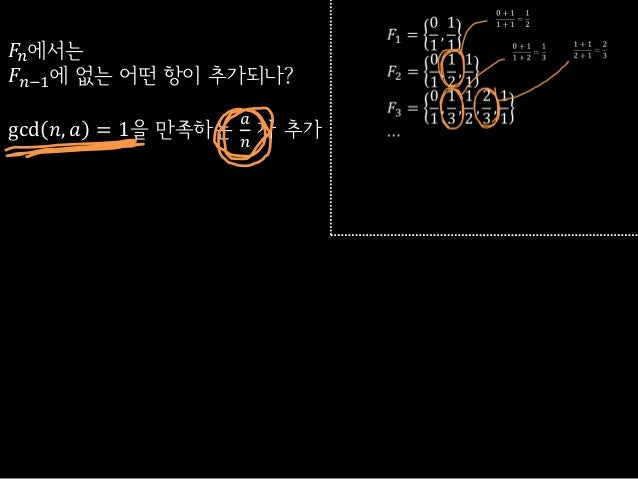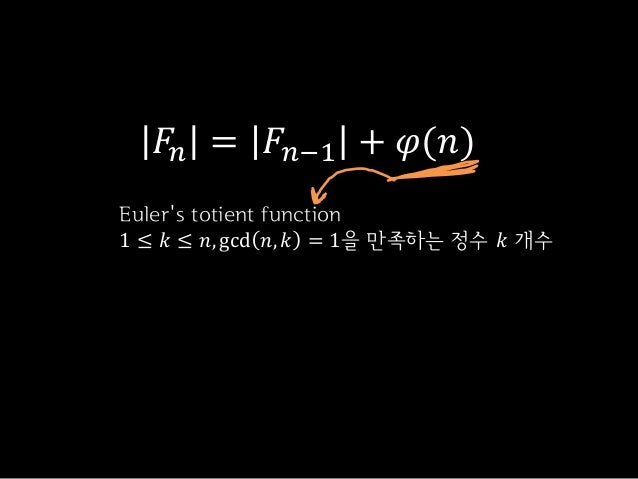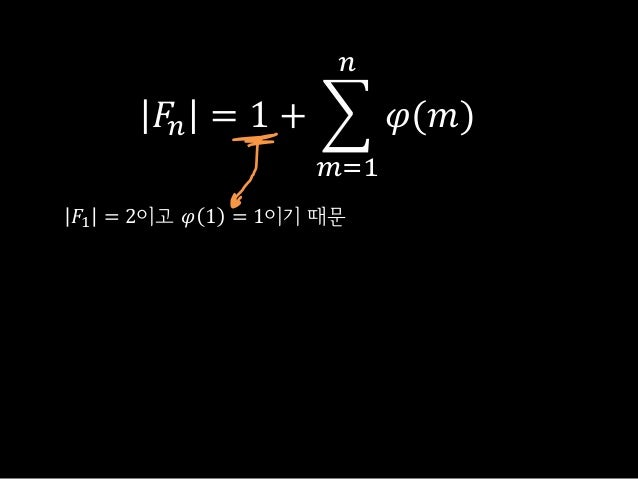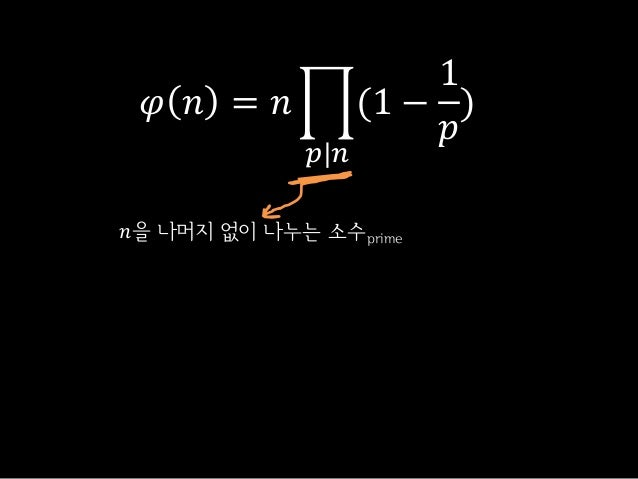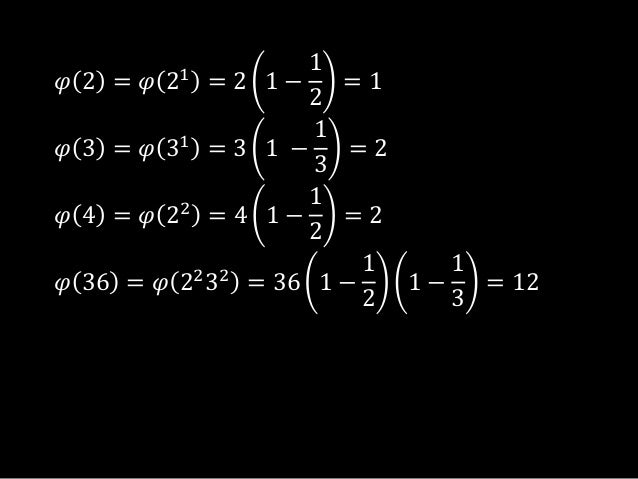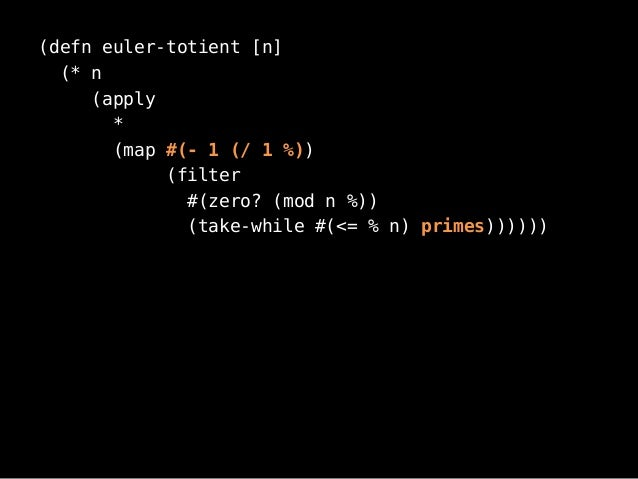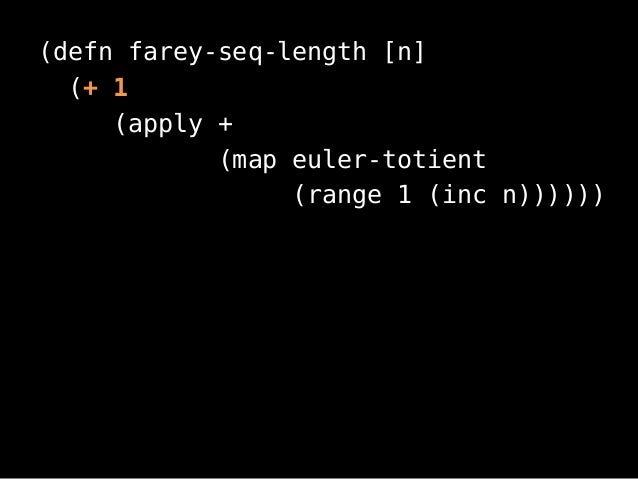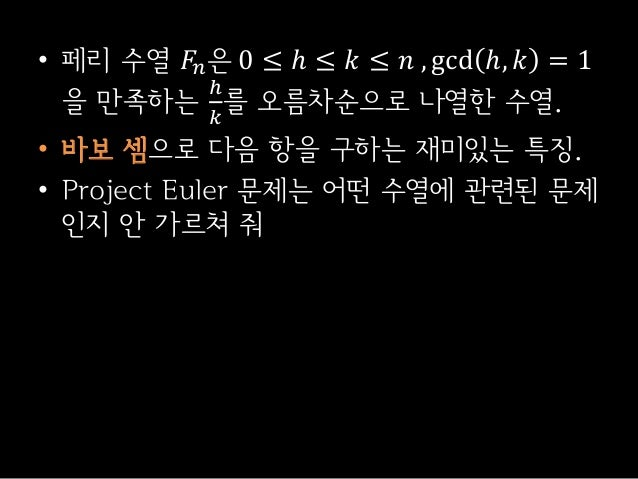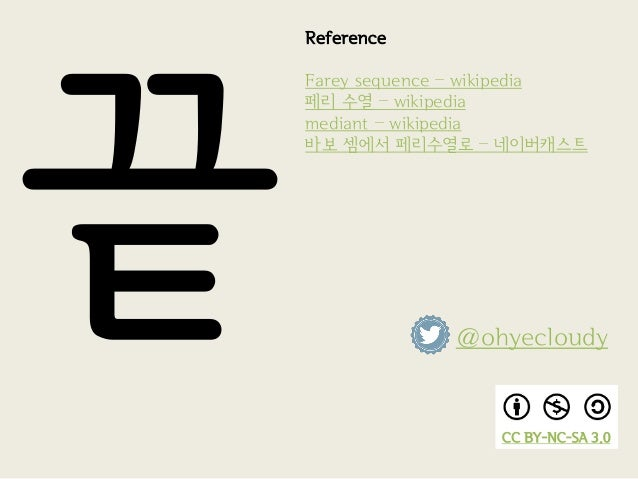Upcoming SlideShare
×
• Full Name
Comment goes here.

Are you sure you want to Yes No• Be the first to comment

### 페리 수열(Farey sequence)

1. 1. 페리 수열 / farey sequence𝐹𝑝𝑟𝑒𝑠𝑒𝑛𝑡𝑖𝑜𝑛 =01,12, … ,페리farey,수열sequence, … ,ohyeohyecloudy,cloudy.com, … ,0 + 11 + 2 ohyecloudy / ohyecloudy.com2013-06-08
2. 2. 어쩌다가 이런 주제로…• Project Euler 삽질이 이끌었다.• 힘으로 푼 뒤 알아 보니 페리 수열
3. 3. 페리 수열 특징을 알아본다.• + 관련된 Project Euler 문제 풀이• 물론 Clojure로
4. 4. 페리 수열?
5. 5. 페리 수열 𝐹𝑛은0 ≤ ℎ ≤ 𝑘 ≤ 𝑛 , gcd ℎ, 𝑘 = 1을만족하는ℎ𝑘를 오름차순으로 나열한 수열
6. 6. 페리 수열 𝐹𝑛은0 ≤ ℎ ≤ 𝑘 ≤ 𝑛 , gcd ℎ, 𝑘 = 1을만족하는ℎ𝑘를 오름차순으로 나열한 수열기약분수irreducible fraction진분수proper fraction
7. 7. 𝐹1 =01,11𝐹2 =01,12,11𝐹3 =01,13,12,23,11𝐹4 =01,14,13,12,23,34,11⋯
8. 8. 어떤 특징을 가진 수열일까?• 기본적인 3가지 특징만 소개• 문제 풀면서 추가로~
9. 9. 𝐹𝑞에서𝑎𝑏<𝑝𝑞<𝑐𝑑인𝑝𝑞는𝑎+𝑐𝑏+𝑑로 구할 수 있다1
10. 10. 𝐹𝑞에서𝑎𝑏<𝑝𝑞<𝑐𝑑인𝑝𝑞는𝑎+𝑐𝑏+𝑑로 구할 수 있다1알고 있는 항member
11. 11. 𝐹𝑞에서𝑎𝑏<𝑝𝑞<𝑐𝑑인𝑝𝑞는𝑎+𝑐𝑏+𝑑로 구할 수 있다1새롭게 추가되는 항이상하게 더하고 있어.
12. 12. 13+12=25• 패기 넘치는 분수 더하기• 바보 셈freshman sum• mediant
13. 13. 𝐹1 =01,11𝐹2 =01,12,11𝐹3 =01,13,12,23,11⋯0 + 11 + 1=120 + 11 + 2=131 + 12 + 1=23
14. 14. 𝐹𝑛은 𝐹𝑛−1을 포함한다.2
15. 15. 𝐹1 =01,11𝐹2 =01,12,11𝐹3 =01,13,12,23,11𝐹4 =01,14,13,12,23,34,11⋯
16. 16. 𝐹1 =01,11𝐹2 =01,12,11𝐹3 =01,13,12,23,11𝐹4 =01,14,13,12,23,34,11⋯
17. 17. 𝑎+𝑐𝑏+𝑑는 𝐹𝑏+𝑑에서 처음으로 나타난다.3
18. 18. 𝑎+𝑐𝑏+𝑑는 𝐹𝑏+𝑑에서 처음으로 나타난다.3분모와 같다
19. 19. 𝐹1 =01,11𝐹2 =01,12,11𝐹3 =01,13,12,23,11𝐹4 =01,14,13,12,23,34,11⋯
20. 20. 기본적인 특징들•𝑎𝑏<𝑝𝑞<𝑐𝑑인𝑝𝑞=𝑎+𝑐𝑏+𝑑• 𝐹𝑛은 𝐹𝑛−1을 포함•𝑎+𝑐𝑏+𝑑는 𝐹𝑏+𝑑에서 처음으로 나타남
21. 21. 이제 문제 풀이• + 풀이에 필요한 추가적인 특징 설명
22. 22. Consider the fraction, 𝑛𝑑, where 𝑛 and 𝑑 are positive integers. If 𝑛 < 𝑑 andHCF 𝑛, 𝑑 = 1, it is called a reduced proper fraction.If we list the set of reduced proper fractions for 𝑑 ≤ 8 in ascending order ofsize, we get:18, 17, 16, 15, 14, 27 , 13, 38, 25, 37, 12, 47 , 35, 58, 23, 57, 34, 45 , 56, 67, 78It can be seen that 25 is the fraction immediately to the left of 37.By listing the set of reduced proper fractions for 𝑑 ≤ 1,000,000 in ascendingorder of size, find the numerator of the fraction immediately to the left of 37.http://projecteuler.net/problem=71ProjectEuler#71
23. 23. 𝐹1,000,000에서37보다 작은 수 중 가장 큰 수를 구하라.
24. 24. 𝐹8 = ⋯ , 25 , 37 , ⋯𝐹12 = ⋯ , 25 , 512 , 37 , ⋯𝐹19 = ⋯ , 512 , 819 , 37 , ⋯…𝐹1,000,000 = ⋯ , 𝑥, 37 , ⋯계산필요 없다
25. 25. (defn rhs-farey-pair [[a b c d]](let [mediant-n (+ a c)mediant-d (+ b d)][mediant-n mediant-d c d]))(rhs-farey-pair [2 5 3 7]) [5 12 3 7]25 , 37512 , 37
26. 26. Consider the fraction, 𝑛𝑑, where 𝑛 and 𝑑 are positive integers. If 𝑛 < 𝑑 andHCF 𝑛, 𝑑 = 1, it is called a reduced proper fraction.If we list the set of reduced proper fractions for 𝑑 ≤ 8 in ascending order ofsize, we get:18, 17, 16, 15, 14, 27 , 13, 38, 25, 37, 12, 47 , 35, 58, 23, 57, 34, 45 , 56, 67, 78It can be seen that there are 3 fractions between 13 and 12.How many fractions lie between 13 and 12 in the sorted set of reduced properfractions for 𝑑 ≤ 12,000?http://projecteuler.net/problem=73ProjectEuler#73
27. 27. 𝐹12,000에서13보다 크고12보다 작은 항의 개수를구하라.
28. 28. 𝐹12,000을 구하려면 𝐹11,999을 구해야 한다.뒤에 붙는 형식이 아니라 중간중간 삽입stack overflow 냄새
29. 29. 바로 다음 항을 구할 수 있나?• 𝑛이 주어졌을 때.
30. 30. 𝐹12,000 = 01 , 112,000 , 𝑥, ⋯이 두 항은 알 수 있다.항상 01 , 1𝑛
31. 31. 𝑎𝑏<𝑐𝑑<𝑝𝑞즉, 이 두 항으로 다음 항을 구하는 방법
32. 32. 𝑎𝑏<𝑐𝑑=𝑎 + 𝑞𝑏 + 𝑞<𝑝𝑞𝒌𝑐𝒌𝑑=𝑎+𝑝𝑏+𝑞를 만족하는 𝒌가 존재𝑐𝑑는 기약분수이기 때문
33. 33. 𝑝𝑞=𝑘𝑐 − 𝑎𝑘𝑑 − 𝑏𝒌𝑐𝒌𝑑=𝑎 + 𝑝𝑏 + 𝑞𝑛
34. 34. max(𝑘) =𝑛 + 𝑏𝑑𝑘𝑑 − 𝑏 ≤ 𝑛𝑘 ≤𝑛 + 𝑏𝑑
35. 35. 𝐹𝑛 =…,𝑎𝑏,𝑐𝑑,𝑘𝑐−𝑎𝑘𝑑−𝑏,…𝑘 =𝑛 + 𝑏𝑑
36. 36. 𝐹4 =01,14,13,12, 𝑥, …𝑎𝑏=13,𝑐𝑑=12, 𝑘 =4+32= 3 𝑝𝑞=23
37. 37. (defn next-term [[a b c d n]](let [k (int (/ (+ n b) d))p (- (* k c) a)q (- (* k d) b)][c d p q n]))(next-term [1 3 1 2 4])[1 2 2 3 4]13,1212,23
38. 38. (defn farey-seq [n](take-while#(<= % 1)(map (fn [[a b _ _ _]] (/ a b))(iterate next-term [0 1 1 n n]))))𝐹2 =01,12,11,32,21, ⋯진분수 가분수improper fraction
39. 39. Consider the fraction, 𝑛𝑑, where 𝑛 and 𝑑 are positive integers. If 𝑛 < 𝑑 andHCF 𝑛, 𝑑 = 1, it is called a reduced proper fraction.If we list the set of reduced proper fractions for 𝑑 ≤ 8 in ascending order ofsize, we get:18, 17, 16, 15, 14, 27 , 13, 38, 25, 37, 12, 47 , 35, 58, 23, 57, 34, 45 , 56, 67, 78It can be seen that there are 21 elements in this set.How many elements would be contained in the set of reduced proper fractionsfor 𝑑 ≤ 1,000,000?http://projecteuler.net/problem=72ProjectEuler#72
40. 40. |𝐹1,000,000| − 2를 구하라.
41. 41. 01,11제외|𝐹1,000,000| − 2를 구하라.
42. 42. 𝐹1,000,000을 구해서 개수를 센다면• 세월아 네월아.• 개수만 빠르게 구할 수 있을까?
43. 43. 𝐹𝑛에서는𝐹𝑛−1에 없는 어떤 항이 추가되나?gcd 𝑛, 𝑎 = 1을 만족하는𝑎𝑛가 추가
44. 44. 𝐹𝑛 = 𝐹𝑛−1 + 𝜑(𝑛)Eulers totient function1 ≤ 𝑘 ≤ 𝑛, gcd 𝑛, 𝑘 = 1을 만족하는 정수 𝑘 개수
45. 45. 𝐹𝑛 = 1 + 𝜑(𝑚)𝑛𝑚=1𝐹1 = 2이고 𝜑 1 = 1이기 때문
46. 46. 𝜑 𝑛 = 𝑛 (1 −1𝑝)𝑝|𝑛𝑛을 나머지 없이 나누는 소수prime
47. 47. 𝜑 2 = 𝜑 21= 2 1 −12= 1𝜑 3 = 𝜑 31= 3 1 −13= 2𝜑 4 = 𝜑 22= 4 1 −12= 2𝜑 36 = 𝜑 2232= 36 1 −121 −13= 12
48. 48. (defn euler-totient [n](* n(apply*(map #(- 1 (/ 1 %))(filter#(zero? (mod n %))(take-while #(<= % n) primes))))))
49. 49. (defn farey-seq-length [n](+ 1(apply +(map euler-totient(range 1 (inc n))))))
50. 50. 마무리
51. 51. • 페리 수열 𝐹𝑛은 0 ≤ ℎ ≤ 𝑘 ≤ 𝑛 , gcd ℎ, 𝑘 = 1을 만족하는ℎ𝑘를 오름차순으로 나열한 수열.• 바보 셈으로 다음 항을 구하는 재미있는 특징.• Project Euler 문제는 어떤 수열에 관련된 문제인지 안 가르쳐 줘
52. 52. CC BY-NC-SA 3.0ReferenceFarey sequence – wikipedia페리 수열 – wikipediamediant – wikipedia바보 셈에서 페리수열로 – 네이버캐스트@ohyecloudy

### Be the first to comment

•#### darklibra

Jun. 8, 2013
•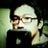#### easthelper

Jun. 8, 2013
•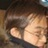#### kaisyu

Jun. 9, 2013
•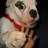#### gwangzingo

Oct. 11, 2014

Total views

8,482

On Slideshare

0

From embeds

0

Number of embeds

4,520

12

Shares

0